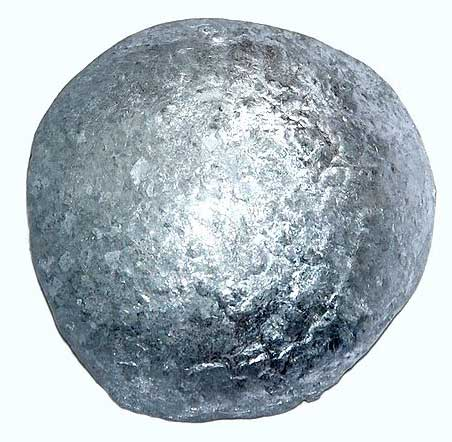10 minutes maximum! Can you do it in 5?

1. A catalyst is a substance that speeds up the rate of a reaction but ...
• A. is chemically unchanged at the end of the reaction.
• B. is chemically changed at the end of the reaction.
• C. does not take part in the reaction.
• D. changes the products made.

2. Catalysts work by ...

• A. giving more particles sufficient energy to react.
• B. increasing the frequency of collisions between reactants.
• C. providing an alternative pathway with lower activation energy.
• D. providing an alternative pathway with higher activation energy.

Q3+4: The diagram below represents the reaction profile for a reaction with and without the catalyst.3. The activation energy for the uncatalysed reaction is represented by the letter ...

4. The activation energy for the catalysed reaction is represented by the letter ...

5. The activation energy for a reaction is ...

• A. the energy supplied to the reactants during a reaction.
• B. the difference in energy between reactants and products.
• C. the minimum energy required by product particles for a reaction to occur.
• D. the minimum energy required by reactant particles for a reaction to occur.

Q6-7: A student investigated the effect on the decomposition of hydrogen peroxide solution of adding metals oxides X, Y, Z and W.

He recorded the volume of oxygen collected in 1 minute and weighed the solid before and after the reaction. His results are recorded below:

 Metal oxide used Volume of oxygen collected in 1 minute / cm3 Mass of metal oxide /g Before reaction After reaction none 0 - - X 82 1 1 Y 158 1 0.6 Z 34 1 1 W 0 1 1

6. Which of the metal oxides acted as catalysts?
• A.    all of them.
• B.    X, Y and Z.
• C.    X and Y.
• D.    X and Z.
7. The most effective catalyst is ...
• A.   metal oxide X.
• B.   metal oxide Y.
• C.   metal oxide Z.
• D.   metal oxide W.

Q8-9: Hydrogen gas is produced when zinc reacts with sulfuric acid. The equation for the reaction is:

Zn(s) + H2SO4(aq)   ZnSO4(aq) + H2(g)

Copper(II)sulfate acts a catalyst in this reaction.
A student decided to investigate if increasing the mass of copper(II)sulfate affected the rate of reaction by recording the volume of hydrogen collected at regular time intervals.8. A suitable method to collect and record the volume of hydrogen would be ...

• A. in a measuring cylinder by the downward displacement of air.
• B. in a measuring cylinder by the upward displacement of air.
• C. in a measuring cylinder by the downward displacement of water.
• D. using an electronic balance.
9. To ensure a fair test the student should ...
• A. repeat the experiment 3 times for each mass of copper(II)sulfate.
• B. use a range of at least 5 different masses of copper(II)sulfate.
• C. use the same mass of zinc and volume of sulfuric acid each time.
• D. repeat the experiment at different temperatures.

10. A biological catalyst is called ...

• A. an inhibitor.
• B. an enzyme.
• C. an isotope.
• D. a substrate.